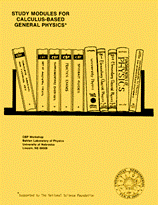## Instructional Materials in Physics and Astronomy## Calculus-Based General Physics

Calculus

#### Date of this Version

1975

From Study Modules for Calculus-Based General Physics
Reproduction rights granted.

#### Abstract

Differentiation of a function, say f(x), is a mathematical operation which yields a second function called the derivative of f [symbolized by f '(x) or dy/dx]. This procedure is represented in the diagram, which shows

Input Differentiation Operation Output

f(x) → f ' (x) = df/dx

the function f(x) being input to an "analytical machine" that manufactures as output the derivative of f. A detailed mathematical prescription for the differentiation operation is nontrivial. It usually involves a quarter or semester course, which requires time, attention, and effort on the student's part.

COinS